#### 相似文章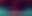# 神经网络变得轻松（第三十四部分）：全部参数化的分位数函数

MetaTrader 5EA交易 | 10 四月 2023, 10:528690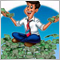### 1. 完全参数化的理论方面

#### 1.1. 隐式分位数网络（IQN）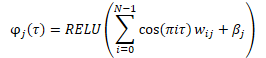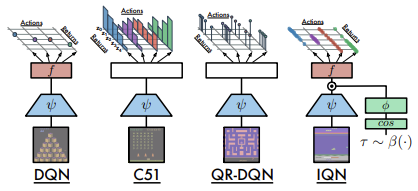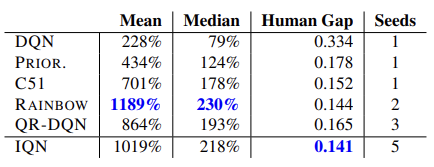#### 1.2. 完全参数化分位数函数（FQF）

2019 年 11 月，提出了分布强化学习的全参数化分位数函数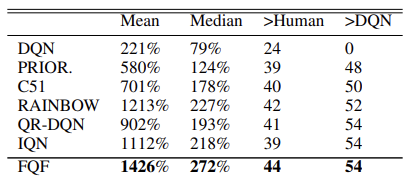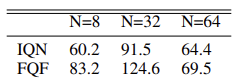### 2. 以 MQL5 实现

```class CNeuronFQF : protected CNeuronBaseOCL
{
protected:
//--- Fractal Net
CNeuronBaseOCL    cFraction;
CNeuronSoftMaxOCL cSoftMax;
//--- Cosine embeding
CNeuronBaseOCL    cCosine;
CNeuronBaseOCL    cCosineEmbeding;
//--- Quantile Net
CNeuronBaseOCL    cQuantile0;
CNeuronBaseOCL    cQuantile1;
CNeuronBaseOCL    cQuantile2;
//---
virtual bool      feedForward(CNeuronBaseOCL *NeuronOCL) override;
virtual bool      updateInputWeights(CNeuronBaseOCL *NeuronOCL) override;

public:
CNeuronFQF();
~CNeuronFQF();
//---
virtual bool      Init(uint numOutputs, uint myIndex, COpenCLMy *open_cl,
uint actions, uint quantiles, uint numInputs,
ENUM_OPTIMIZATION optimization_type, uint batch);
//---
virtual bool      Save(int const file_handle) override;
virtual bool      Load(int const file_handle) override;
//---
virtual int       Type(void) override        const { return defNeuronFQF; }
virtual CLayerDescription* GetLayerInfo(void) override;
};

```

• numOutputs — 下一层中的神经元数量
• myIndex — 层中当前神经元的索引
• open_cl — 指向所操控 OpenCL 设备的对象指针
• actions — 可能的代理者动作数量
• quantiles — 分位数
• numInputs — 前一个神经层的大小
• optimization_type — 优化模型参数所用的函数
• batch — 参数更新的批量大小

```bool CNeuronFQF::Init(uint numOutputs, uint myIndex, COpenCLMy *open_cl,

uint actions, uint quantiles, uint numInputs,
ENUM_OPTIMIZATION optimization_type, uint batch)
{
if(!CNeuronBaseOCL::Init(numOutputs, myIndex, open_cl, actions, optimization, batch))
return false;
SetActivationFunction(None);

```

```//---
if(!cFraction.Init(0, myIndex, open_cl, actions * quantiles, optimization, batch))
return false;
cFraction.SetActivationFunction(None);
//---
if(!cSoftMax.Init(0, myIndex, open_cl, actions * quantiles, optimization, batch))
return false;
cSoftMax.SetActivationFunction(None);

```

```   if(!cCosine.Init(numInputs, myIndex, open_cl, actions * quantiles, optimization, batch))
return false;
cCosine.SetActivationFunction(None);
//---
if(!cCosineEmbeding.Init(0, myIndex, open_cl, numInputs, optimization, batch))
return false;
cCosineEmbeding.SetActivationFunction(LReLU);

```

```   if(!cQuantile0.Init(4 * actions * quantiles, myIndex, open_cl, numInputs, optimization, batch))
return false;
cQuantile0.SetActivationFunction(None);
//---
if(!cQuantile1.Init(actions * quantiles, myIndex, open_cl,
4 * actions * quantiles, optimization, batch))
return false;
cQuantile1.SetActivationFunction(LReLU);
//---
if(!cQuantile2.Init(0, myIndex, open_cl, actions * quantiles, optimization, batch))
return false;
cQuantile2.SetActivationFunction(None);
//---
return true;
}

```

#### 2.1. 前馈```__kernel void FQF_Cosine(__global float* softmax,
__global float* output)
{
size_t i = get_global_id(0);
size_t total = get_global_size(0);
size_t action = get_global_id(1);
int shift = action * total;
//---
float result = 0;
for(int it = 0; it < i; it++)
result += softmax[shift + it];
result += softmax[shift + i] / 2.0f;
output[shift + i] = cos(i * M_PI_F * result);
}

```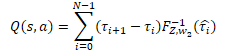```__kernel void FQF_Output(__global float* quantiles,
__global float* delta_taus,
__global float* output,
uint total)
{
size_t action = get_global_id(0);
int shift = action * total;
//---
float result = 0;
for(int i = 0; i < total; i++)
result += quantiles[shift + i] * delta_taus[shift + i];
output[action] = result;
}

```

```bool CNeuronFQF::feedForward(CNeuronBaseOCL *NeuronOCL)
{
if(!cFraction.FeedForward(NeuronOCL))
return false;
if(!cSoftMax.FeedForward(GetPointer(cFraction)))
return false;

```

```     {
uint global_work_offset = {0, 0};
uint global_work_size;
global_work_size = Output.Total();
global_work_size = cSoftMax.Neurons() / global_work_size;
OpenCL.SetArgumentBuffer(def_k_FQF_Cosine, def_k_fqf_cosine_softmax, cSoftMax.getOutputIndex());
OpenCL.SetArgumentBuffer(def_k_FQF_Cosine, def_k_fqf_cosine_outputs, cCosine.getOutputIndex());
if(!OpenCL.Execute(def_k_FQF_Cosine, 2, global_work_offset, global_work_size))
{
printf("Error of execution kernel FQF_Cosine: %d", GetLastError());
return false;
}
}

```

```   if(!cCosineEmbeding.FeedForward(GetPointer(cCosine)))
return false;

```

FQF 算法的下一步中，我们必须将当前系统状态（初始数据）的嵌入与分位数嵌入相结合。 如您所忆，对于此操作，我们决定使用 Dropout 神经层内核。 在这个内核的主体中，我们针对 40 个元素向量运用了向量操作。 由此，线程数将比数据缓冲区的大小少四倍。

```     {
uint global_work_offset = {0};
uint global_work_size = {(cCosine.Neurons() + 3) / 4};
OpenCL.SetArgumentBuffer(def_k_Dropout, def_k_dout_input, NeuronOCL.getOutputIndex());
OpenCL.SetArgumentBuffer(def_k_Dropout, def_k_dout_map, cCosineEmbeding.getOutputIndex());
OpenCL.SetArgumentBuffer(def_k_Dropout, def_k_dout_out, cQuantile0.getOutputIndex());
OpenCL.SetArgument(def_k_Dropout, def_k_dout_dimension, (int)cCosine.Neurons());
if(!OpenCL.Execute(def_k_Dropout, 1, global_work_offset, global_work_size))
{
printf("Error of execution kernel Dropout: %d", GetLastError());
return false;
}
}

```

```   if(!cQuantile1.FeedForward(GetPointer(cQuantile0)))
return false;
//---
if(!cQuantile2.FeedForward(GetPointer(cQuantile1)))
return false;

```

• 定义任务空间
• 将指向缓冲区的指针和其它必要信息传递给内核参数
• 调用内核执行过程

```     {
uint global_work_offset = {0};
uint global_work_size = { Neurons() };
OpenCL.SetArgumentBuffer(def_k_FQF_Output, def_k_fqfout_quantiles, cQuantile2.getOutputIndex());
OpenCL.SetArgumentBuffer(def_k_FQF_Output, def_k_fqfout_delta_taus, cSoftMax.getOutputIndex());
OpenCL.SetArgumentBuffer(def_k_FQF_Output, def_k_fqfout_output, getOutputIndex());
OpenCL.SetArgument(def_k_FQF_Output, def_k_fqfout_total,
(uint)(cQuantile2.Neurons() / global_work_size));
if(!OpenCL.Execute(def_k_FQF_Output, 1, global_work_offset, global_work_size))
{
printf("Error of execution kernel FQF_Output: %d", GetLastError());
return false;
}
}
//---
return true;
}

```

#### 2.2. 反馈

```__kernel void FQF_OutputGradient(__global float* quantiles,
__global float* delta_taus,
__global float* output_gr,
__global float* quantiles_gr,
__global float* taus_gr
)
{
size_t i = get_global_id(0);
size_t total = get_global_size(0);
size_t action = get_global_id(1);
int shift = action * total;

```

```   float gradient = output_gr[action];
quantiles_gr[shift + i] = gradient * delta_taus[shift + i];
taus_gr[shift + i] = gradient * quantiles[shift + i];
}

```

```__kernel void FQF_QuantileGradient(__global float* state_embeding,
__global float* taus_embeding,
__global float* quantiles_gr,
__global float* state_gr,
__global float* taus_gr
)
{
size_t i = get_global_id(0);
size_t total = get_global_size(0);
size_t action = get_global_id(1);
int shift = action * total;
//---
float gradient = quantiles_gr[shift + i];
state_gr[shift + i] = gradient * taus_embeding[shift + i];
taus_gr[shift + i] = gradient * state_embeding[shift + i];
}

```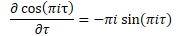• Softmax 层结果
• 所获误差梯度
• 结果缓冲区 — SoftMax 层结果缓冲区级别的误差梯度

```__kernel void FQF_CosineGradient(__global float* softmax,
__global float* output_gr,
__global float* softmax_gr
)
{
size_t i = get_global_id(0);
size_t total = get_global_size(0);
size_t action = get_global_id(1);
int shift = action * total;

```

```   float cumul = 0;
for(int it = 0; it < i; it++)
cumul += softmax[shift + it];

```

```   float result = -M_PI_F * i * sin(M_PI_F * i * (cumul + softmax[shift + i] / 2)) * output_gr[shift + i];

```

```   for(int it = i + 1; it < total; it++)
{
cumul += softmax[shift + it - 1];
float temp = cumul + softmax[shift + it] / 2;
result += -M_PI_F * it * sin(M_PI_F * it * temp) * output_gr[shift + it] *
softmax[shift + it] / temp;
}
softmax_gr[shift + i] += result;
}

```

```bool CNeuronFQF::calcInputGradients(CNeuronBaseOCL *NeuronOCL)
{
return false;

```

```     {
uint global_work_offset = {0, 0};
uint global_work_size = { cSoftMax.Neurons() / Neurons(), Neurons() };
cQuantile2.getOutputIndex());
cSoftMax.getOutputIndex());
{
printf("Error of execution kernel FQF_OutputGradient: %d", GetLastError());
return false;
}
}

```

```   if(!cQuantile1.calcHiddenGradients(GetPointer(cQuantile2)))
return false;
return false;

```

```     {
uint global_work_offset = {0, 0};
uint global_work_size = { cCosineEmbeding.Neurons(), 1 };
NeuronOCL.getOutputIndex());
cCosineEmbeding.getOutputIndex());
{
printf("Error of execution kernel FQF_OutputGradient: %d", GetLastError());
return false;
}
}

```

```   if(!cCosine.calcHiddenGradients(GetPointer(cCosineEmbeding)))
return false;

```

```     {
uint global_work_offset = {0, 0};
uint global_work_size = { cSoftMax.Neurons() / Neurons(), Neurons() };
cSoftMax.getOutputIndex());
{
printf("Error of execution kernel FQF_CosineGradient: %d", GetLastError());
return false;
}
}

```

```   if(!cSoftMax.calcInputGradients(GetPointer(cFraction)))
return false;
//---
return true;

```

```bool CNeuronFQF::updateInputWeights(CNeuronBaseOCL *NeuronOCL)
{
if(!cFraction.UpdateInputWeights(NeuronOCL))
return false;
if(!cCosineEmbeding.UpdateInputWeights(GetPointer(cCosine)))
return false;
if(!cQuantile1.UpdateInputWeights(GetPointer(cQuantile0)))
return false;
if(!cQuantile2.UpdateInputWeights(GetPointer(cQuantile1)))
return false;
//---
return true;
}

```

```class CFQF : protected CNet
{
private:
uint              iCountBackProp;
protected:
uint              iUpdateTarget;
//---
CNet              cTargetNet;
public:
CFQF(void);
CFQF(CArrayObj *Description)  { Create(Description); }
bool              Create(CArrayObj *Description);
~CFQF(void);
bool              feedForward(CArrayFloat *inputVals, int window = 1, bool tem = true)
{ return        CNet::feedForward(inputVals, window, tem); }
bool              backProp(CBufferFloat *targetVals, float discount = 0.9f,
CArrayFloat *nextState = NULL, int window = 1, bool tem = true);
void              getResults(CBufferFloat *&resultVals);
int               getAction(void);
int               getSample(void);
float             getRecentAverageError() { return recentAverageError; }
bool              Save(string file_name, datetime time, bool common = true)
{ return CNet::Save(file_name, getRecentAverageError(), (float)iUpdateTarget, 0, time, common); }
virtual bool      Save(const int file_handle);
virtual bool      Load(string file_name, datetime &time, bool common = true);
//---
virtual int       Type(void)   const   {  return defFQF;   }
virtual bool      TrainMode(bool flag) { return CNet::TrainMode(flag); }
virtual bool      GetLayerOutput(uint layer, CBufferFloat *&result)
{ return        CNet::GetLayerOutput(layer, result); }
//---
virtual void      SetUpdateTarget(uint batch)   { iUpdateTarget = batch; }
virtual bool      UpdateTarget(string file_name);
};

```

```bool CFQF::backProp(CBufferFloat *targetVals, float discount = 0.9f,
CArrayFloat *nextState = NULL, int window = 1, bool tem = true)
{
//---
if(!targetVals)
return false;

```

```   if(!!nextState)
{
vectorf target;
if(!targetVals.GetData(target) || target.Size() <= 0)
return false;
if(!cTargetNet.feedForward(nextState, window, tem))
return false;
cTargetNet.getResults(targetVals);
if(!targetVals)
return false;
target = target + discount * targetVals.Maximum();
if(!targetVals.AssignArray(target))
return false;
}

```

```   if(iCountBackProp >= iUpdateTarget)
{
#ifdef FileName
if(UpdateTarget(FileName + ".nnw"))
#else
if(UpdateTarget("FQF.upd"))
#endif
iCountBackProp = 0;
}
else
iCountBackProp++;

```

```   return CNet::backProp(targetVals);
}

```

```int CFQF::getAction(void)
{
CBufferFloat *temp;
CNet::getResults(temp);
if(!temp)
return -1;
//---
return temp.Maximum(0, temp.Total());
}

```

```int CFQF::getSample(void)
{
CBufferFloat* resultVals;
CNet::getResults(resultVals);
if(!resultVals)
return -1;

```

```   vectorf temp;
if(!resultVals.GetData(temp))
{
delete resultVals;
return -1;
}
delete resultVals;
//---
if(!temp.Activation(temp, AF_SOFTMAX))
return -1;
temp = temp.CumSum();

```

```   int err_code;
float random = (float)Math::MathRandomNormal(0.5, 0.5, err_code);
if(random >= 1)
return (int)temp.Size() - 1;
for(int i = 0; i < (int)temp.Size(); i++)
if(random <= temp[i] && temp[i] > 0)
return i;
//---
return -1;
}

```

### 3. 测试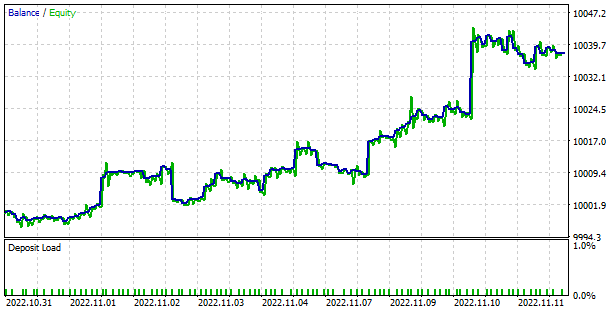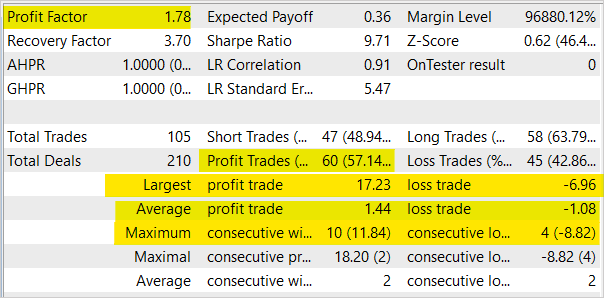### 本文中用到的程序

# 发行 类型 说明
1 FQF-learning.mq5 EA 优化模型的 EA
2 FQF-learning-test.mq5 EA

3 FQF.mqh  类库 FQF 模型类
4 NeuroNet.mqh 类库 创建神经网络模型的类库
5 NeuroNet.cl 代码库

NetCreator.mq5 EA 模型构建工具
7 NetCreatotPanel.mqh  类库 创建工具的类库

MQL5.zip (106.93 KB)

#### 该作者的其他文章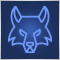种群优化算法：灰狼优化器（GWO）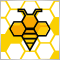种群优化算法：人工蜂群（ABC）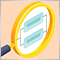构建自动运行的 EA（第 08 部分）：OnTradeTransaction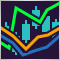非线性指标